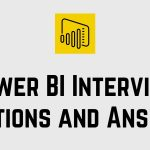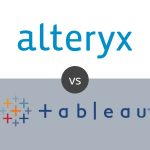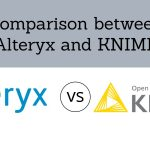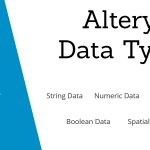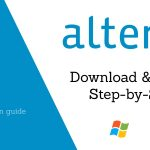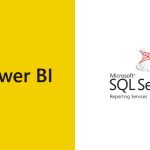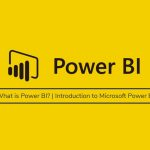[email protected] +1 203-349-9909 / +91-8080042523

Excel Function

### How to use Clean Function in Excel

The CLEAN Function in Excel takes a text string and returns a text that has been “cleaned” of line breaks and other non-printable characters. What is a CLEAN Function? What is the syntax for CLEAN Function? How to use CLEAN Function? And finally, What are the applications of CLEAN Function? ➡ What is Clean Function in...

### How to use the Excel Choose Function

The CHOOSE Function in Excel returns a value from a list using a given position or index. The values provided to CHOOSE can include references. Let’s have a look below to get more details about CHOOSE Function. ➡ What is Excel Choose Function? CHOOSE Function is a function in Excel that helps you to get...

### How to use the Excel Code Function

The CODE Function in Excel returns a numeric code for a given character. The CODE Function can be used to understand numeric codes given to the CHAR Function as it is the reverse process of CHAR Function. ➡ What is Code Function in Excel? The Function that accepts one argument, for the text for which you...

### How to use the Excel Column Function

The COLUMN function in Excel returns the column number for a reference. In other words, Column Function too will return the column number of the cell which contains formula when reference is not provided. ➡ What is Column Function in Excel? The function which will returns column number for a reference is called Excel COLUMN Function....

### How to use Ceiling Function in Excel

The CEILING Function in Excel returns a given number rounded up to a specified multiple. CEILING Function is always used for rounding up. Its purpose is to Round a number up to nearest multiple. It returns a rounded number. What is a CEILING Function in Excel? What is the syntax for CEILING Function? Where can...

### How to use Char Function in Excel

The CHAR Function in Excel returns a character when given a valid Character Code. CHAR Function is used to specify the characters that are difficult to enter in a formula in Excel. Now, let us study in detail the CHAR Function as given below. What is a CHAR Function in Excel? What is the formula...

### How to use ABS Function in Excel

An Excel Function is a predefined formula that executes the actions like calculating values in a precise order. The common functions of Excel are AGGREGATE, AVERAGE, COUNT, INDEX, ROUND, SUM etc. In this blog, we are going to see all the Excel Functions one by one in depth. Also, you will learn the different parts of a function and how to...

### How to use AND Function in Excel

An Excel Function is a predefined formula that executes the actions like calculating values in a precise order. The common functions of Excel are AGGREGATE, AVERAGE, COUNT, INDEX, ROUND, SUM etc. In this blog, we are going to see all the Excel Functions one by one in depth. Also, you will learn the different parts of a function and how to...